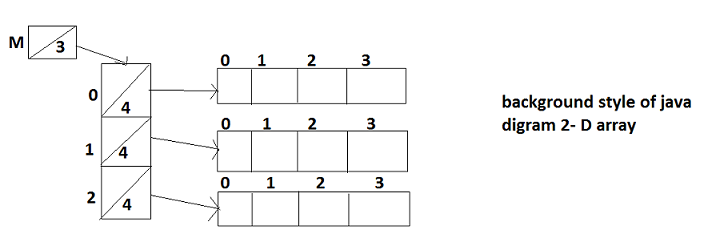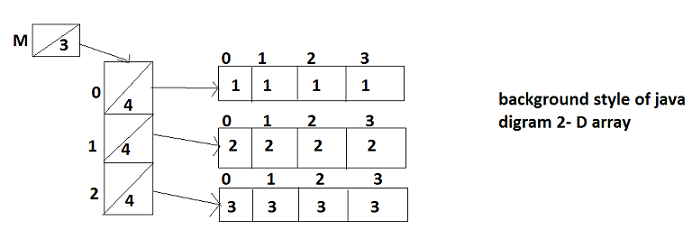# Java Programing laungage

Two Dimensional Array
 Previous Home Next

A two-dimensional array is an array in with both rows and columns. Multi dimensional arrays are arrays of arrays. You can create arrays of two or more dimensions.

1. Declare multi dimensional array :
2. Syntax :

`type array-Name[][];`

Where type defines the data type of array element like int, double etc.

array-name is the name of array.

Note: that the two sets of brackets indicates that there are two dimensions for this array.

Example :

`int m[][]; //m is the array reference variable`
3. Allocation Memory for its Elements
4. Syntax :

`array-Name = new type[size1][size2];`

Where array-Name is the name of the array and type is a valid java type and dimensions are specified by size1 and size2 in the array.

Example :

`m= new int;`
5. Structure of Multi Dimensional Array
6.Note:That array indexes begins with zero. That means if you want to access 1st element of an array use zero as an index.

Example :

```int m[][]=new int[r][c];

or

int [][]m=new int;

or

int m[][]={{1,1,1,1},{2,2,2,2},{3,3,3,3};``````System.out.print(m.length); // ans= 3

System.out.print(m.length); // ans= 4

System.out.print(m); // ans= 2

System.out.print(m); // errorrrrr```
 Previous Home Next